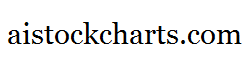Members Area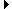Sign Up | Log In
 Free Level 2 QuotesStock AnalysisCorrelation Trading PlatformCorrelationsMembership Benefits

P2RANGE
 Lookup a different indicator.

 Indicator Usage Description Example p2range p2range[n] OPERATOR (INDICATOR or POSITIVE NUMBER) , where n is a positive integer >= 0. Returns the percentage change from the lowest price among day n and day n-1 to the highest price among day n and day n-1 where n=0 is the last trading day, n=1 is the previous day, etc. (p2range < 3)

Introduction:

The 'p2range' indicator is used in indicator formula construction to narrow the stock pre-screener results to include only those stocks that have a percentage change from the lowest to highest price of 2 consecutive days that are restricted to the parameters set for the p2range indicator.

Example:

The chart of Intel (INTC) below highlights days where the percentage change from the lowest to highest stock price of 2 consecutive days was less than 3%.

 Chart of Intel (INTC) highlighting days where the percentage change from the lowest to highest price of 2 consecutive days was less than 3%.

INDICATORS IN OUR DATABASE THAT INCLUDE THE P2RANGE INDICATOR...

Click here to view all indicators that include the indicator 'p2range' (sorted by AISCORE)

 (p2range > 10)AISCORE: 2962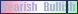Indicator ID: 060130212312(p2range < 3)AISCORE: 2403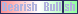Indicator ID: 060419204700(close < sma) and (close > sma) and (vol > 1.05 * vol) and (p2range < 4)AISCORE: 1730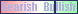Indicator ID: 060519102712(close < sma) and (close > sma) and (vol > 1.05 * vol) and (p2range < 5)AISCORE: 1695Indicator ID: 060519102743(close < sma) and (close > sma) and (vol > 1.05 * vol) and (p2range < 6)AISCORE: 1649Indicator ID: 060519102835 More ->